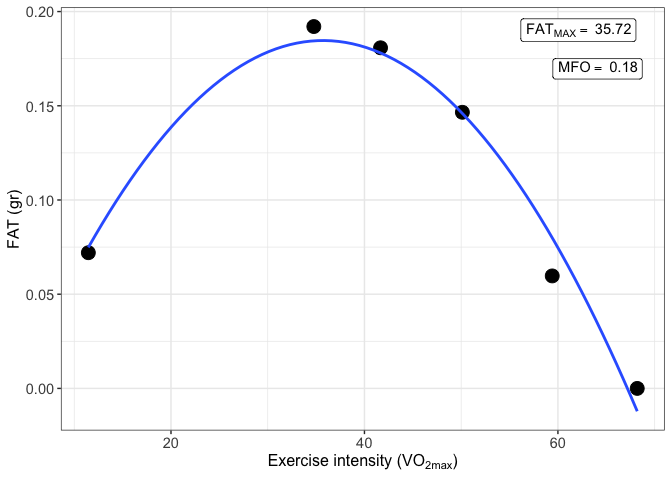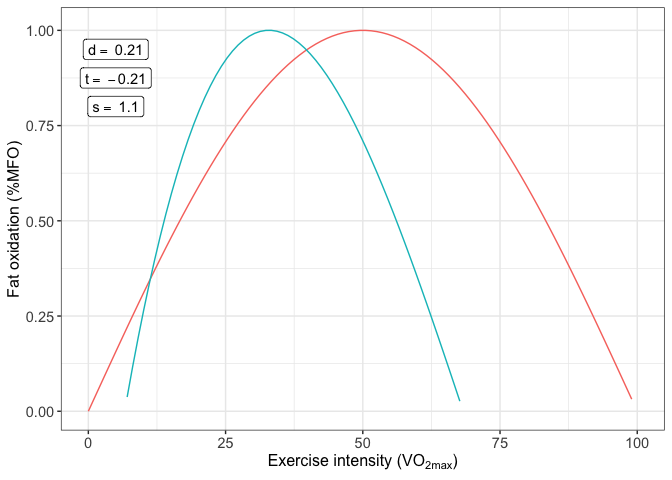# MFO

## Overview

The MFO package have been designed to calculate the Maximal Fat Oxidation (MFO), the exercise intensity that elicits MFO (Fatmax) and the SIN model to represent the fat oxidation kinetics. Three variables can be obtained from the SIN model: dilatation (d), symmetry (s) and traslation (t).Additionally, the package allows to calculate MFO and Fatmax of multiple subjects.

## Example

This is a basic example which shows you how to use the MFO package:

``````library(devtools)
install_github("JorgeDelro/MFO")
library(MFO)``````

First, we have to load the data which consists in 3 databases: - basal_df: basal metabolism database. - MFO_df: MFO test database. - VO2max_df: results of a graded exercise test of which the VO2max of the subject is going to be extracted.

``````
data(list = c("basal_df", "MFO_df", "VO2max_df"), package = "MFO")
# Convert to data.frame
basal_df <- data.frame(basal_df)
MFO_df <- data.frame(MFO_df)
VO2max_df <- data.frame(VO2max_df)``````

Then, we can used the function MFO

``````result_MFO <- MFO(step_time = 20,
db_MFO = MFO_df,
db_basal = basal_df,
cv_var = "RER",
author = "Frayn",
VO2max = NULL)``````

and the MFO can be plotted

``print(result_MFO\$MFO_plot)``MFO kinetics are calculated using a database returned from MFO function called MFO_db.

``result_MFO_kinetics <- MFO_kinetics(result_MFO\$MFO_db)``

And again the function returns a plot with the results calculated

``print(result_MFO_kinetics\$MFO_kinetics_plot)``